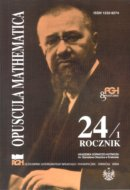Opuscula Math. 24, no. 1 (2004), 19-33

Opuscula Mathematica

# Discretization of the stationary distribution of heat in the non-homogeneous body

Bogusław Bożek

Abstract. We give a short survey on the theory of the mixed boundary-value problem for the stationary Fourier equation in a non-homogeneous medium defined on any Lipschitz domain $$\Omega\subset\mathbb{R}^n$$ ($$n\geq 2$$). The compatibility condition for the thermal flux has been established by the standard procedure of integration the divergence.

Keywords: elliptic partial differential equations, stationary distribution of heat, discretization method.

Mathematics Subject Classification: 35J25, 35J20, 35J67, 65N30, 41A65.

Full text (pdf)

• Bogusław Bożek
• AGH University of Science and Technology, Faculty of Applied Mathematics, al. Mickiewicza 30, 30-059 Cracow, Poland